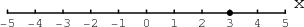# Math Insight

### Image: Point in one dimensionThe graph of the equation $x=3$ is a point if it is an equation in one dimension.

Image file: point_in_1D.png